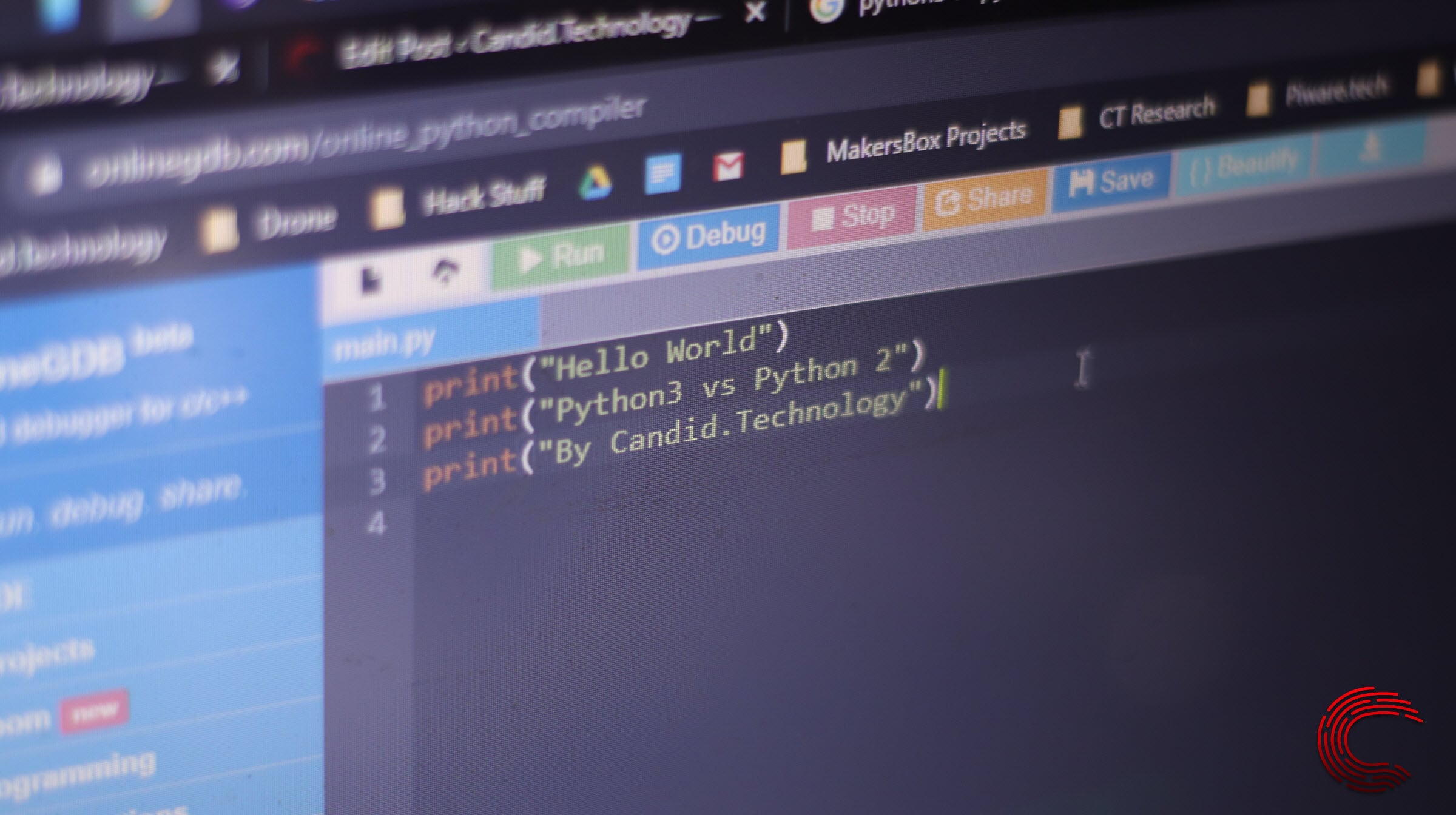# How to use the Python range() function?Python’s range function works exactly what it sounds like; it generates a range of numbers between the given starting and endpoints. If the programmer wants, they can also add a step argument. The function is generally used along with the for loop to iterate over a sequence of numbers.

However, the function works differently in Python 2 and Python 3. In Python 2, the range() function gives a range of numbers between two specified points in the form of a list. The xrange() function also does the same, except it returns an xrange object rather than a list.

When dealing with Python 3, there’s no xrange()  function to worry about. The range() function behaves like the xrange() function from Python 2 and returns a sequence of integers between two mentioned points with the mentioned increments in a range object.

# Python range() basic syntax

The basic syntax for the range() function is as follows.

``range(start, stop[, steps])``

Note that you can run the command with either one, two or three arguments depending on what you want the function to achieve.

Do keep in mind that the arguments provided to the range constructor should be integers. Floating, decimal or any other type of number aren’t allowed.

Also read: Bash While loop explained

# Python range with one parameter

The required parameter for range() in Python 3 is stop. This means that you have to at the very least specify the exit of the range.

``````for i in range(5):
print(i)``````

The above example will print a sequence of integers from zero to four with increments of one. When you use this command with only the stop parameter, the command automatically picks zero as a starting point and increments with one.

If the passed argument is zero or an incorrect number, the function returns an empty sequence.

Also read: How to install Python on Windows?

# Python range with two parameters

As you’d probably have guessed, when working with the start and stop parameters, the function generates a sequence between the start and end points with increments of one in between,

``````for i in range(3, 5):
print(i)``````

The above example will only print 3 and 4 because that’s the range we’ve specified in the parameters. Do keep in mind that the stop parameter should be greater than start; otherwise, you’ll get an empty list. In this case, you can use zero and negative integers as arguments.

Also read: How to create Bash aliases?

# Python range with three parameters

If you provide all three parameters, the function will give you a sequence of integers following the rules specified. Do keep in mind that if the step parameter is positive, the range will increase successively, and if it’s negative, you’ll get the opposite result.

``````for i in range(0, 26, 5):
print(i)``````

The above example will print a sequence from 0 to 25 with increments of five in between. Make sure that the stop parameter is greater than the start one.

Similarly, if we take this the other way around and the step parameter is negative, we’ll get a decreasing sequence. Once again, make sure that the start parameter is greater than the stop parameter; otherwise, you’ll end up with an empty sequence.

``````for i in range(20, 4, -5):
print(i)``````

The above snippet will output a sequence starting from 20, going all the way down to 5 with increments of 5 in between.

If you pass zero as the step value, you’ll get a ValueError exception.

Also read: How to install pip in Ubuntu?